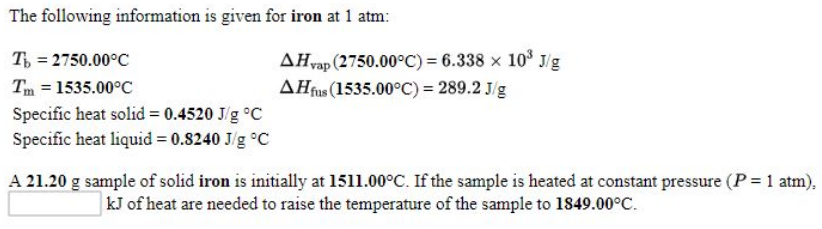# Problem: he following information is given for iron at 1 atm: Tb = 2750.00°C        ΔHvap (2750.00°C) = 6.338 x 103 J/gTm = 1535.00°C        ΔHfus (1535.00°C) = 289.2 J/gSpecific heat solid = 0.4520 J g°C Specific heat liquid = 0.8240 J g°C   A 21.20 g sample of solid iron is initially at 1511.00°C. If the sample is heated at constant pressure (P = 1 atm).________kJ of heat are needed to raise the temperature of the sample to 1849.00°C.

###### FREE Expert Solution
92% (377 ratings)###### Problem Details

he following information is given for iron at 1 atm:

Tb = 2750.00°C        ΔHvap (2750.00°C) = 6.338 x 103 J/g

Tm = 1535.00°C        ΔHfus (1535.00°C) = 289.2 J/g

Specific heat solid = 0.4520 J g°C

Specific heat liquid = 0.8240 J g°C

A 21.20 g sample of solid iron is initially at 1511.00°C. If the sample is heated at constant pressure (P = 1 atm).

________kJ of heat are needed to raise the temperature of the sample to 1849.00°C.Frequently Asked Questions

What scientific concept do you need to know in order to solve this problem?

Our tutors have indicated that to solve this problem you will need to apply the Heating and Cooling Curves concept. You can view video lessons to learn Heating and Cooling Curves. Or if you need more Heating and Cooling Curves practice, you can also practice Heating and Cooling Curves practice problems.

What professor is this problem relevant for?

Based on our data, we think this problem is relevant for Professor Chandrasekhar's class at FAU.